﻿ The Experimental Teaching of the Conics Using the Reflection of the Light: The Conceptual Study of the Cassegrain TelescopePublications are Open
Access in this journal
Article Versions
Export Article
• Normal Style
• MLA Style
• APA Style
• Chicago Style
Original Article
Open Access Peer-reviewed

The Experimental Teaching of the Conics Using the Reflection of the Light: The Conceptual Study of the Cassegrain Telescope

Juan Carlos Ruiz Mendoza
American Journal of Educational Research. 2019, 7(10), 699-707. DOI: 10.12691/education-7-10-5
Received August 15, 2019; Revised October 03, 2019; Accepted October 18, 2019

Abstract

The study of conics has to get applications in science, particularly in Astronomy. So the objective of this work is to demonstrate the reflective properties of the hyperbola and the parabola and its relationship with the conceptual study of a Cassegrain telescope from the perspective of Geometry Optics. For this purpose, an optical prototype was designed using low-cost materials. An instructional guide will also be provided to prove that the reflective proprieties of these conics are fulfilled by light rays.

1. Introduction

To reduce the gap between teaching and learning the author takes into account the findings of Alborch, Benegas, and Pandiella 1, as described in a paper they have published after the World Conference of Physics and Sustainable Development (WCPSD). The Conference approved a document that proposes various actions to promote and improve the teaching of Physics, including a strong recommendations to prepare teaching materials and organizing professional teacher training workshops, for physics teacher trainers in different regions of the developing world 2.

On the other hand, the United Nations Education, Science and Culture Organization (UNESCO) promotes actions for the active learning of the Physics where the student carries out experimental activities and new methods and strategies to interest and motivate them.

Pérez, Lozada y Falcón 3 outlined there is not a divorce between the theory and the laboratory activities but a close relationship between them. That is, the experiments that are carried out at the laboratory can be used as a resource in the classroom to understand the theory, and training scientific thinking capacities. Such link between theory and practice has been a teaching principle for professors that pursued students’ understanding of theoretical concepts, their ability to recognize the application of scientific knowledge in everyday objects as well as their capacity to recognize physical phenomena as normal events. Conducting experiments in the classroom fosters the development of competencies for the students’ scientific education in a social context 4.

Another source of information 5 outlines that in building the scientific knowledge, the experimental activities are not a priority in the teaching-learning process due to the lack of laboratories, limited training of professors and the lack of practical activities suggested in the junior and senior high school textbooks. Consequently, few alternatives for analyzing, scientific querying, discussion and scientific interpretation of physical phenomena are left out.

Yuri Surin 6 has declared that making experiments in the classroom is a tool for students’ development of competencies of observation, hypotheses testing; as well as for fostering interest and habits for the study of sciences, among other benefits.

Rivero 7 suggests that well-planned demonstrative experiments under the teacher guidance should never be forgotten, so that the students may realize that Physics is a natural science and that organized practical activity might be intended for the searching of information to understand the surrounding world, and for learning to learn by facing reality with good sense and definite criterion. Such is the fundamental task of a challenging professor seeking students’ motivation.

As Analytic Geometry (particularly the study of the conics) is concerned, a large number of authors of different countries makes reference to its study in a geometric and analytic way, and propose pedagogic strategies for its learning just as Garzón 8, Alegría 9 and Pérez 10, among others, do. The study of the conics using the reflection properties reports extensive information of its applications in the fields of the sciences, such as Physics, Medicine or Architecture (particularly in the Optical Geometrics Optics). However, the use of demonstrative experiments using light-reflecting properties as a teaching procedure is still to be explored and described. The use of light-reflective properties has been left to the specialists in the optic design and the laboratories specialized in the construction of the optic components of spherical and aspherical surfaces for its use in optic instruments as the telescopes, photographic cameras, microscopes, etc.

It is well known that there are companies specialized in designing and selling laboratory equipment for the teaching of sciences. However, their products are so expensive that educational institutions cannot afford such prices. As a result, the teaching process relies upon explaining the theory and solving exercises, the students never complete experimental activities connecting theory to practice 11.

The connection of theory to practice is a means for the adequate understanding of theory as the interpretation of natural processes. Relating theoretical instruction to practical activities, under the teaching guidance, favors students’ learning 12. Therefore, considering the didactic significance of connecting theory to practice, and the high prices of laboratory equipment, the author designed and construct an optical prototype using ordinary non-expensive raw material and components. This prototype was intended for the study of conics (hyperbola and parabola) by applying the reflective properties of light.

2. Materials and Methods

An optical prototype was designed for conducting experiments in the teaching of conics. In assembling this equipment low-cost materials were used. The author prepared a user’s manual explaining how to operate the optical prototype.

• Figure 1. Geometric Optics prototype for the study of conics

The Geometry Optics prototype for the study of conics (Figure 1) consists of an incandescent bulb (light source), two spherical lenses having different focal distances, a light beam collecting table, three grids to simulate parallel beams (one having three divisions, another having only two division and a third one having no divisions), one parabola component, two hyperbola component, three parabola and hyperbola combining components having different focal distances, and an spherical component (aimed to reflect light beams in the same direction). These components are used for the conceptual demonstration of the conics using light reflection laws, illustrating the functioning of the Cassegrain classical telescope.

The following is a description of the components of the parabola, the hyperbola, the spherical components and the representation of the Cassegrain telescope. These components were made up of 1mm thick acrylic, cut with a laser where a semi-transparent material is placed to represent the surfaces of the conics reflecting and transmitting light (Figure 2, Figure 3, and Figure 4).

• Figure 2. Acrylic components of the parabola where the slide is placed
• Figure 3. Cassegrain telescope slide components placed above the surface
• Figure 4. Acrylic components used for reflecting light

The activity that is carried out with this experimental equipment (Figure 1) was designed for the teaching of conics or for teaching a course of Geometrics Optics. The prototype is a material aid that can be used in the classroom as a means to illustrate and prove theoretical notions 13. The workshop resulting from the use of the prototype would have a demonstrative and interactive character and the students are expected to be as active as if they were working at real-time in a lab.

To monitor the experimental introduction of the prototype, the concepts of hyperbola and parabola, as well as the definitions of lights reflection and focal distance of the converging lens are presented to the students.

3. Results

3.1. Geometric Notions to be Taught

The definitions of the hyperbola and the parabola were taken from Lehmann’s Analytic Geometry 14. Lehmann defines the hyperbola as follows: “A hyperbola is the geometric place of the points such that the absolute value of the difference of their distances to two fixed points of the plane, called foci (F, F'), is always equal to a constant, positive amount and less than the distance between the focuses” (Figure 5).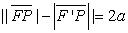• Figure 5. The hyperbola representation

Hyperbola elements:

1. Foci: They are the fixed points F and F'.

2. Main or real axis: It is the line that passes through the foci.

3. Secondary or imaginary axis: It is the mediatrix of the FF' segment

4. Center: It is the point of intersection of the axes.

5. Vertexes: Points A and A' are the points of intersection of the hyperbola with the focal axis. Points B and B' they are obtained as intersection of the imaginary axis with the circumference that has for center one of the vertexes and radius c.

6. Radius vectors: They are the segments that go from a point of the hyperbola to the foci: PF and PF '.

7. Focal Distance: It is the segment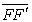of length 2c.

8. Major axis: It is the segmentof length 2a.

9. Minor axis: It is the segment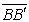of length 2b.

10.Axes of symmetry: They are the lines of the real axis or the imaginary axis.

11.Asymptote: they are the lines representing the equations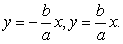(1)

12.Relation between semi-axes: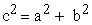(2)

Equilateral hyperbola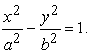(3)

13.The straight side (SS) is the segment Q Q’, its ends are points of the curve, perpendicular to the focal axis crossing one of the focus. The corresponding equation is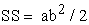(4)

14.The eccentricity is defined as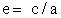(5)

But, if the hyperbole c> a, then e > 1.

The axis mentioned above might be indistinctively of different or equal length, in any case the hyperbola will be horizontal. Their magnate will depend of the larger or lesser opening of the hyperbola branches.

3.1.1. The Parabola

A parabola is a geometric place of a point that moves in a plane in such a way that the distance from a fixed line located at the plane is always equal at the distance from a fixed point of the plane out of that line. That fixed point is called focus and the straight line directrix of the parabola.

The description of a parabola when the vertex is at the origin and its axis coincides with one of the coordinate axes is illustrated in Figure 6.

• Figure 6. Description of the parabola

Let us designate focal axis of the parabola to the line that goes by F and is perpendicular to D. Let us represent the point V (0,0), as the midpoint of the DF segment, or point where the parabola intersects the focal axis.

The coordinates of the focus F (P, 0) which is the point that is located on the focal axis and the distance that lies from the vertex to the focus is equal to the distance between the vertex and the directrix. The D directrix D (- p, 0) is the perpendicular line to the axis of the parabola being at the same distance from the vertex as the focus vertex.

The line segment that joins two points of the parabola is called a chord; In particular, a focal chord perpendicular to the axis is called the straight side.

Let P (x, y) be any point of the parabola. For P (x, y) we trace the A segment perpendicular to D as shown in the figure, then, by the definition of the parabola, the P point must satisfy the geometric condition 1FP1 = 1PA1

Hence the following equation is obtained: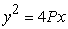(6)

The equation of the parabola with vertex at the x axis as the axis of the parabola

P = Focal distance magnitude.

3.1.2. Laws of Reflection of Light and Focal Length of a Converging Lens

According to Freedman, Sears, and Zemansky 15 the laws of reflection are defined as follows (Figure 7): 1st. Law: The incident ray, the reflected ray and the normal one are on the same plane. 2nd. Law: The angle of incidence is equal to the angle of reflection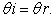(7)
• Figure 7. Law of the reflection of light

According to the law of reflection, a beam obliquely striking the surface originates an angle with the tangent of the surface equal to the coming out beam (Figure 8). The same is true for the reflective properties of conics.

• Figure 8. Reflection of light when striking at conic surface (parabola, ellipsis, hyperbola)

3.1.3. Focal Distance of Converging Lens

In Smith’s book 16 the converging lensare defined as an arrangement of at least two surfaces that, when striking parallel rays at an angle to the focal axis of the lens, they will cross a point of the focal plane, but if these rays are parallel to the focal axis of the lens, as shown in Figure 9, they will cross a point called the lens focus.

• Figure 9. Focal distance of converging lens

3.1.4. Reflective Properties of Conics from the Perspective of Geometric Optics

When studying optical equipment light is considered as beams striking transparent surfaces like spherical and aspherical mirrors as conics that according to the reflective properties of the hyperbola and the parabola ensure that:

1. If we consider the branches of the hyperbola to be a mirror, the beams that strike the surface of one of the branches in the direction of one the foci will be reflected upon the its surface towards the other focus (Figure 10 & Figure 11).

• Figure 10. Beams reflected towards the other focus of the hyperbola
• Figure 11. Beams directed towards the focus of one of the branches of the hyperbola and reflected to the other focus

2. The beams coming out from one of the foci of a hyperbola branch when striking any branch (taken as mirrors) will be reflected moving further causing the impression that they are coming from the other focus (Figure 12).

• Figure 12. Beams coming from the focus of the hyperbola and reflected to the other branch of the hyperbola as if they were coming from the branch of this branch

3. The beams crossing the focus of a parabola will be reflected parallel to its axis of symmetry. Conversely, the beams coming from the infinite, as a very distant source reaching the parabola and parallel to its axis of symmetry, will cross the focus when reflected (Figure 13, Figure 14)

• Figure 13. Beams coming out of the focus and reflected on the surface parallel to the axis
• Figure 14. Parallel beams to the optical axis reflected a surface towards the focus of the parabola

Some of these reflecting properties of conics will be experimentally proved with the optical prototype, in addition to combining the optical properties of the parabola and hyperbola for a conceptual illustration of Cassegrain telescope operation by explaining the laws of light 17.

Here we describe the classic Cassegrain telescope (Figure 15 and Figure 16), which consists of two mirrors: a parabolic primary mirror and a hyperbolic secondary mirror where beams coming from the infinite strike parallel to the surface of the parabolic primary mirror. These rays will be directed towards their focus according to the reflexive properties of the parabola described above. By interposing a hyperbolic secondary mirror (a branch of the parabola) having a focus that coincides with that of the parabola, the reflected beams will cross through the other focus of the branch of the hyperbola where an instrument is placed to collect those beams to observe a distant object like a star or a planet.

• Figure 15. Cassegrain classic telescope
• Figure 16. Cassegrain telescope drawing
3.2. Experimental Demonstration

Next, with the proposed optical equipment, the reflective properties of these conics described above and the conceptual operation of the classic Cassegrain telescope will be experimentally explained by means of the light reflection laws.

3.2.1. Parallel rays

With the experimental equipment and its corresponding instruction manual, the following arrangement is possible (Figure 17), where three parallel beams are obtained, and subsequently, a converging lens is interposed to locate its focal length that matches the explained theory of the geometric optics for converging lenses (Figure 18).

• Figure 17. Experimental arrangement to obtain parallel light beams on the collection table
• Figure 18. Parallel beams crossings or converging in the focus of the biconvex lens

3.2.2. Geometric Optics Demonstration of the Reflexivity of the Hyperbola and Parabola

The experimental arrangement previously described will be used to demonstrate the reflexivity of the hyperbola and parabola. A converging lens is placed at the component of the hyperbola described in the optical equipment of the conics, parallel beams are led to cross the lens so that to converge with its focus. As illustrated in Figure 19, the lens is placed to make the focus coincides with one of the branches of the hyperbole, then a semi-reflective surface is placed on its branch (surface that partly allows to reflect and transmit light rays) in such a way that beams crossing the surface follow the same direction towards the focus, whereas those that are reflected cross through the other focus of the other branch of the hyperbola, Figure 20 and Figure 21) as predicted by the theory of reflexivity of the hyperbola and the law of reflection of light.

• Figure 19. Beams converging in one of the focus of the hyperbola
• Figure 20. Beams reflected towards the other focus of the hyperbola
• Figure 21. A beam of light directed to one focus of the hyperbola and reflected towards the other focus of the hyperbola

Another experimental arrangement of reflection of the hyperbola may be the following: Let parallel beams strike and cross through a converging lens (of a focal distance of 10cm) and make the focus of this lens to coincide with the focus of one of the branches of the hyperbola. The converging lens is located on the left side of the other branch of the hyperbola in such a way that a reflective semi-transparent surface is placed on this branch of the hyperbola and it is observed that the beams are partly transmitted in the same direction towards the focus of the branch of the hyperbola and partially reflected towards the focus of the other branch of the hyperbola as seen in Figure 22.

• Figure 22. Beams directed to the focus of one of the branches of the hyperbola and beams reflected to the other focus of the hyperbola

The theory also predicts that the beams coming out from one of the foci of one of the branches of the hyperbola when reaching the other branch are reflected moving away from the surface as if they were coming from the focus of that branch.

For the corresponding experimental demonstration, the following experiment is carried out: At the first arrangement, where parallel beams were obtained using a converging lens that interrupts the parallel beams and making them converge on the lens focus. The hyperbola component is placed where the focus of the converging lens coincides with the focus of one of the branch of the hyperbola, and those rays are made to reach the next surface of the branch of the hyperbola (Figure 23), where a semitransparent one millimeter surface lamella is placed in such a way that the beams are reflected and transmitted almost in the same direction, the refraction is difficult to observe because of small thickness of the lamella (Figure 24).

• Figure 23. Beams coming out of the focus of one branch of the hyperbola and reaching the other branch of the hyperbola
• Figure 24. Beams reflected and crossing through the semitransparent surface

What we want to demonstrate is that the beams reflected in the branch of the hyperbola when moving away from its surface looks as if they were coming from the focus of that branch. Recalling the previous experiment, where beams directed to the focus of one of its branches when interposing a semitransparent lamella on the branch of the hyperbola were reflected towards the focus of the interposed branch.

Coming back to the experiment illustrated in Figure 24, where beams transmitted on the surface branch, return in the same direction (according to the laws of reflection of light on spherical surfaces), when placing the spherical component (explained in Figure 4), we are now considering the spherical mirror as a source of light that emits these rays towards the focus of the hyperbola branch; as the example in Figure 22, the light source (mirror spherical) is on the right side of another branch of the hyperbola, in such a way that a reflective semi-transparent surface is placed on this branch of the hyperbola; it is observed that the light rays are partly transmitted in the same direction towards the focus of the branch of the hyperbola and the reflected ones of the branch interposed towards the focus of this branch (Figure 25), where these rays are in the same direction of the reflected rays of Figure 24. That is according to the principle of reversibility of optical paths, if a light beam propagates following a path, that path is not modified if the direction of propagation is reversed.

• Figure 25. Beams coming from the spherical surface and reflecting on the branch of the hyperbola interposed towards its focus

Several examples are presented where the beams coming from the focus strike the reflective branch of the hyperbola in different positions (Figure 26 and Figure 27), backing up the theory of light reflexivity on hyperbolic surfaces.

• Figure 26. The direction of beams reflected by the interposed branch towards the focus
• Figure 27. Beams reflecting on the branch interposed towards its focus

3.2.3. Experimental Arrangement to Explain the Concept of the Cassegrain Telescope

The Cassegrain telescope (Figure 16 and Figure 17) is composed of two conical surfaces, the parabola, and hyperbola and other optical systems, where distant objects are observed. To demonstrate the explained concept of the Cassegrain telescope, a parabola and hyperbola component was designed so that the focus of the parabola coincides with one of the foci of the hyperbola and the other focus coincides with the vertex of the parabola (Figure 3).

Before demonstrating the concept of the Cassegrain telescope, let's demonstrate the concept of the parabola described in the reflection properties. According to the fifth property, parallel beams when striking the reflecting surface of the parabola crosses its the focus. For this purpose, the experimental arrangement illustrated in Figure 2 was designed, where the experimental equipment makes parallel beams (Figure 17) to strike its focal axis (axis of symmetry that passes through the focus, the vertex, and is perpendicular to D directrix). When reflected on the surface of the parabola, they effectively cross its focus, those backing up the theory as shown in Figure 28.

• Figure 28. Beams reflecting on the surface of the parabola and crossing its focus

That fifth property of the reflection also states that beams crossing through the focus when reflected by the surface of the parabola come out in the parallel direction. However, as the manual of the optical prototype explains, the equipment was designed so as to lead the reflected beams to come out parallel to their focal axis, see Figure 29.

• Figure 29. Beams coming out of the focus of the parabola and reflecting parallel to the focal axis

The experimental demonstration of the concepts behind the Cassegrain telescope is recommended using the materials shown in Figure 3. To carry out the experiment an arrangement to obtain parallel light beams on the collection table is needed (Figure 17). Two parallel beams striking the collecting table should be obtained, then the Cassegrain telescope component is placed, where these beams are parallel to the focal axis of the telescope (or symmetrically perpendicular to its surfaces), two semi-transparent lamellae are placed there, one on the surface of the parabola and the other in one of the branches of the hyperbola, which according to the design, the focus of the parabola coincides with one of the foci of a branch of the hyperbola. As observed in the experiments (Figure 30 and Figure 31) the light beams that strike the parabola are reflected to the branch of the hyperbola which in turn are reflected to the vertex of the parabola. The idea behind the telescope designed is illustrated proving the theory validity.

• Figure 30. The Cassagrain telescope physical concept representation
• Figure 31. The Cassagrain telescope physical concept representation
• Figure 32. Beams directed to the vertex of the parabola
• Figure 33. Beams directed to focus of the parabola

Other experimental arrangements of the reversibility of the light beams designed for the Cassegrain telescope component are shown, where one of the foci of the hyperbola coincides with the focus of the parabola as shown in Figure 32, Figure 33 and Figure 34) and explained at the equipment manual.

• Figure 34. Beams reflecting on the surface of the parabola and crossing its focus and beams reflecting on the surface of the branch of the hyperbola and crossing one of its foci
• Figure 35. Beams reflected towards the vertex of the parabola composing the telescope

Figure 35 is an experimental arrangement resembling the design of the Cassegrain telescope. The lamella of the reflective surface is placed on the branch of the surface that is closest to the surface of the parabola, and as it has been shown that any beam directed to one of the foci of the hyperbola when reflected on one of its surfaces either will cross through the focus or will be reflected, as if it were coming from the focus of the branch reflecting it according to the definitions of reversibility of the conics explained previously.

4. Conclusion

The equipment designed is a powerful teaching aid to the professor that teaches Analytic Geometry or Geometric Optics. It will foster theory understanding and relates theory to practice as a didactic tool for the learning of conics. The equipment may be constructed using non-expensive materials, its construction only demands to have a sound knowledge of Geometric Optics.

References

  Alborch, A., Benegas, J., Pandiella, S., “Enseñanza de la Óptica Geométrica en las escuelas secundarias usando la estrategia ALOP”, Revista de Enseñanza de la Física, 28 (extra), 179-186, November. 2016. In article  UNESCO, World Conference of Physics and Sustainable Development (WCPSD) Final Declaration, [Online]. http://www.wcpsd.org/education/ [Accessed Dic. 10, 2014]. In article  Pérez, E., Falcón, N, “Diseño de prototipos experimentales orientados al aprendizaje de la óptica”, Revista Eureka sobre Enseñanza y Divulgación de las Ciencias, 6 (3), 452-465,2009. In article View Article  Hodson, D., “Hacia un enfoque más crítico del trabajo de laboratorio”, Perfiles Educativos, 23 (94), 299-313, 2001. In article  García, R., “Las actividades experimentales en la escuela secundaria”, Perfiles Educativos, 23 (94), 70-90, 2001. In article  Surin, Y., Tres conferencias sobre metodología de la enseñanza de la Química. Editorial de Libros para la Educación, 1981. In article  Rivero, H., Cómo mejorar mi clase de Física en el nivel superior. Editorial Trillas, Mexico, 2004. In article  Garzón, M., Propuesta didáctica para la enseñanza de las propiedades de reflexión de las cónicas por medio de la metodología de resolución de problemas, Universidad Nacional de Colombia, Bogotá, 2003. In article  Alegría, P. (2014). Las cónicas y sus aplicaciones, UNAM, Mexico, [Online]. http://www.ehu.es/~mtpalezp/conicas.pdf [Accessed Dic. 10, 2014]. In article  Pérez, G., Estudio de las aplicaciones de las cónicas mediado por la modelación desde una visión analítica. Universidad Nacional de Colombia, Bogotá, 2012. In article  Solbes, J., Zacarés J., ¿Qué sucede con la enseñanza de la óptica? Revista Española de Física 7(4) 38-43, 1993. In article  Bellot, N., Cantero, Z., Lozada, G., Menéndez, P., Meléndez, A, L., “El experimento demostrativo en las clases de ciencias naturales de secundaria básica: una variante metodológica para su desarrollo y perfeccionamiento”,Ensaio Pesquisa em Educaçãoem Ciência, 9 (2), 2007, pp. 1-15, 2007. In article View Article  Sokoloff, D. R., Thornton, R. K., Laws, P. W., Real Time Physics Third Edition, Wiley, New Jersey, 2012. In article  Lehmnn, C. H., Geometría Analítica, Editorial LIMUSA, SA, Mexico, 2008. In article  Freedman, R. A., Sears, F., Zemansky, M. W., Física universitaria, Pearson Educación, Mexico, 2009. In article  Smith, W. J., Modern Optical Engineering: The Design of Optical Systems, Second Edition, McGraw-Hill, New York, 1990. In article  see http://www.telescopios.org/tienda/telescopios_reflectores.htm and 6b taken from the source arquimedes.matem.unam.mx/PUEMAC/PUEMAC_2008/conicas…hipérbola.html). In articleThis work is licensed under a Creative Commons Attribution 4.0 International License. To view a copy of this license, visit http://creativecommons.org/licenses/by/4.0/